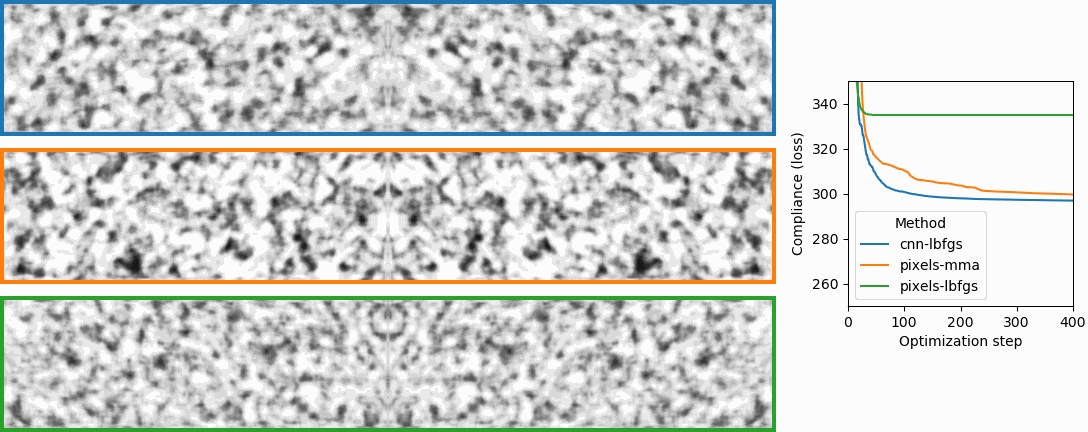Figure 1: Optimizing a bridge structure. In the top frame, optimization happens in the weight space of a CNN. In the next two frames it happens on a finite element grid.

## A Visual Introduction

In this post we propose using neural networks to reparameterize physics problems. This helps us design better bridges, skyscrapers, and cantilevers while enforcing hard physical constraints. In the figure above, you can see that our approach optimizes more quickly and has a smoother transition from large-scale to small-scale features. In the figure below, you can explore all 116 tasks that we studied.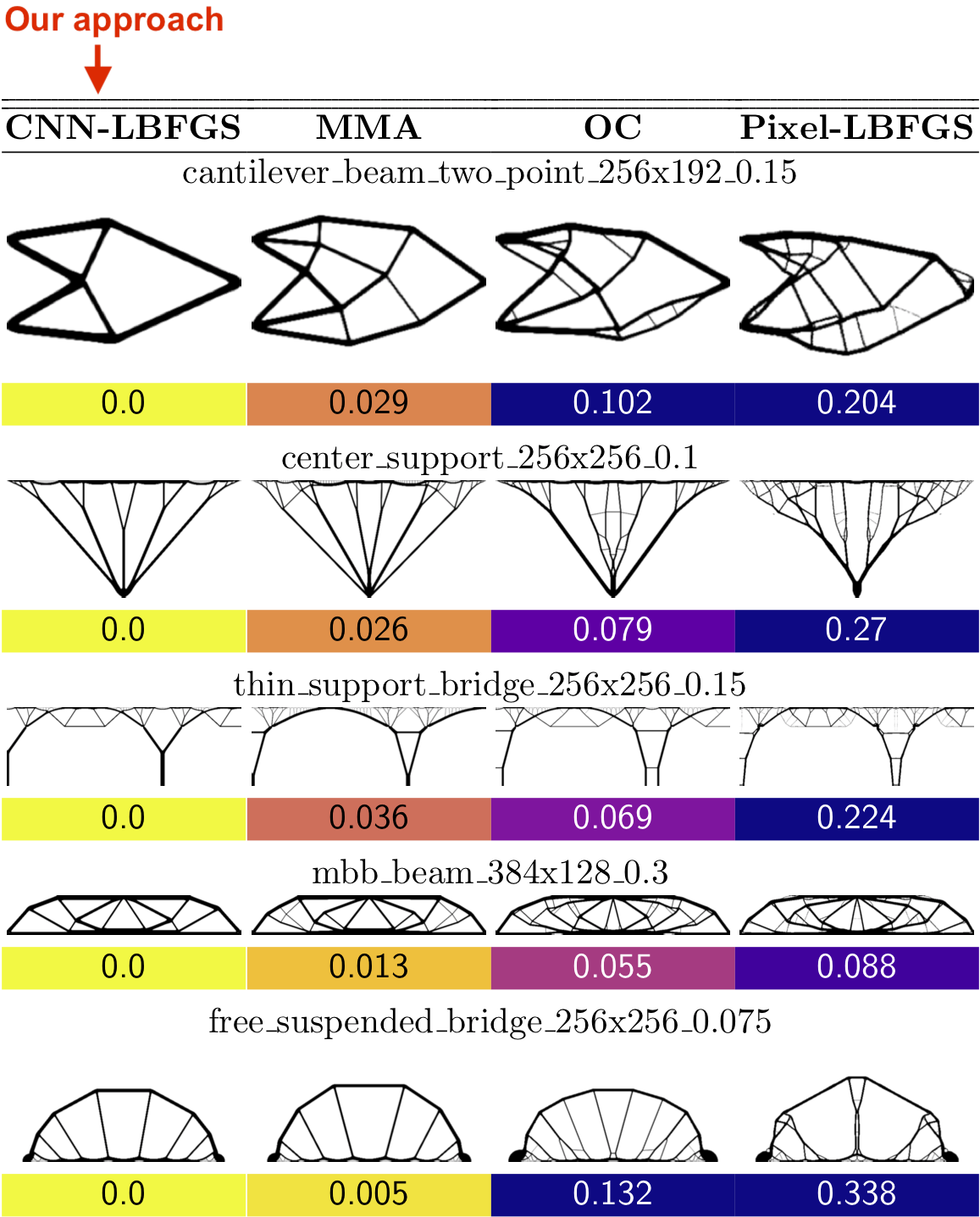Figure 2: Results from the 116 structural optimization tasks. The scores below each structure measure how much worse the design was than the best overall design.

Now that I’ve sparked your curiosity, I’m going to use the rest of this post to put our results in the proper context. The proper context is parameterization and my message is that it matters much more than you might expect.

## A Philosophical Take on Parameterization

The word “parameterization” means different things in different fields. Generally speaking, it’s just a math term for the quirks and biases of a specific view of reality. Consider, for example, the parameterizations of a 3D surface. If the surface is rectilinear, then we’d probably want to use Cartesian coordinates. If it’s cylindrical or spherical, we may be better off using polar or spherical coordinates. So we have three parameterizations but each one describes the same underlying reality. After all, a sphere will remain a sphere regardless of how its equation is written.

Reparameterization. And yet, some parameterizations are better than others for solving particular types of problems. This is why reparameterization – the process of switching between parameterizations – is so important. It lets us take advantage of the good properties of two different parameterizations at the same time. For example, when we are editing a photograph in Photoshop, we may edit specific objects while working in a pixel parameterization. Then we may switch to a Fourier basis in order to adjust lighting and saturation. Technically speaking, we’ve just taken advantage of reparameterization.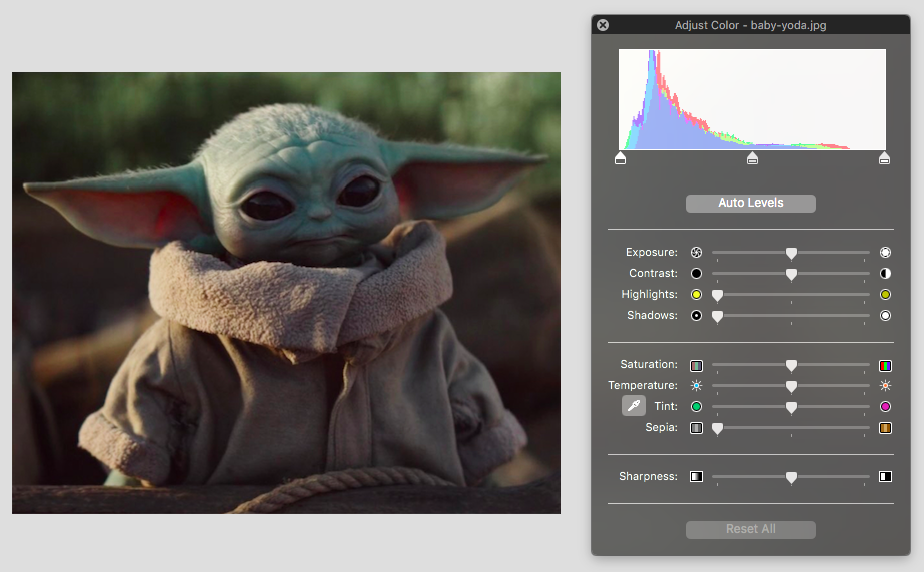Figure 3: A simple example of reparameterization. We might use the pixel image of Baby Yoda (on the left) to make spatially localized edits. Then we might use its spectral decomposition (on the right) to adjust lighting and saturation.

Physics examples. Physicists use reparameterization or “change of basis” tricks all the time. Sometimes it’s a matter of notation and other times it’s a matter of what question they are asking. Here are a few examples:

These are some of the simplest examples, but there are countless others. In this post we’ll focus on an exciting new tool for doing this sort of thing: neural networks.

## Reparameterization and Neural Networks

Neural networks have all sorts of nice properties. They work well with high-dimensional data, they have great spatial priors, and they can change their representations during learning. But there is still a lot that we don’t understand about them. In fact, two recent studies suggest that we’ve underestimated the importance of their architectural priors.

The Deep Image Prior.1 The first study tells us that even untrained networks have fantastic image priors. The authors hammer this point home by showing that it’s possible to perform state-of-the-art denoising, super-resolution, and inpainting on a single image with an untrained network.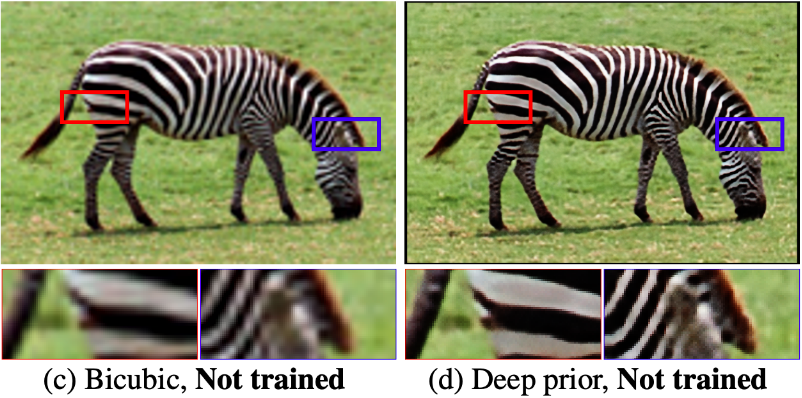Figure 4: Visualization of the Deep Image Prior. Optimizing a single image using the weight space of an untrained CNN gives state of the art super-resolution results.

Differentiable Image Parameterizations2. The second study highlights the relationship between image parameterizations and better optimization results. The authors argue that well-chosen parameterizations can

• Precondition the optimization landscape
• Enforce constraints (such as conservation of volume)
• Bias optimization towards certain outcomes
• Implicitly optimize other objects (eg a 3D surface projected to 2D)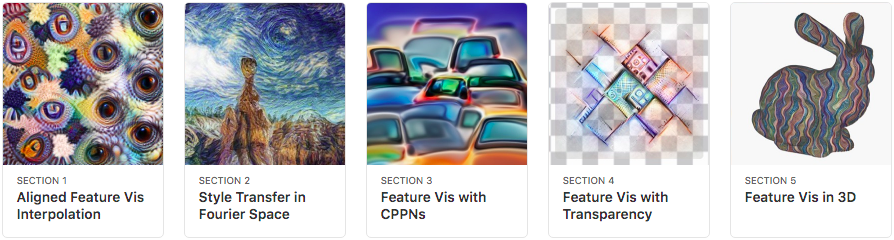Figure 5: Specific examples of differentiable image parameterizations applied to neural network visualizations and art.

These two papers are interesting because they don’t focus on the process of training neural networks. Rather, they focus on how this process is shaped by good priors.

## Good Priors for Physics

With the rise of powerful neural network models, many scientists have grown interested in applying them to physics research. But there are some challenges. Data that is generated by physical processes is subject to exact physical constraints such as conservation of energy, mass, or charge and it can be challenging to enforce these constraints on neural network models. Another challenge is that neural networks require large datasets which are not always practical in physics. So the core challenge is to leverage deep learning…

• without sacrificing exact physics (many people train models to approximate physics, but often that isn’t enough)
• without excessively large training datasets (often we only care about a few solutions)

Satisfying these requirements with supervised learning methods is hard. But what if we used neural networks for reparameterization instead? When we looked into this idea, we found lots of evidence that the deep image prior extends beyond natural images. Some examples include style transfer in fonts3, uncertainty estimation in fluid dynamics4, and data upsampling in medical imaging5. Indeed, whenever data contains translation invariance, spatial correlation, or multi-scale features, the deep image prior is a useful tool. So we decided to push the limits of this idea in the context of physics. We chose structural optimization as a case study because it’s a domain where good spatial priors are essential.

## The Joys of Structural Optimization

Structural optimization is a computational tool which, in an ironic turn of events, often comes up with more organic-looking structures than human engineers do. These structures are beautiful, lightweight, and extremely strong.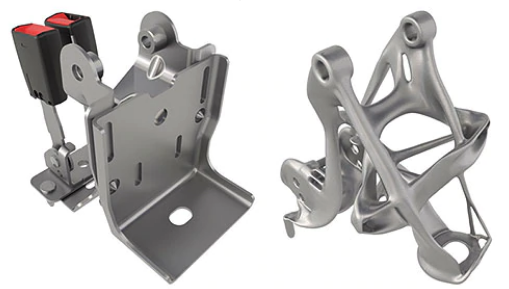Figure 6: Autodesk uses structural optimization to augment the design process.

How it works. In structural optimization, you are given a fixed amount of material, a set of anchor points, and a set of force points. Your goal is to design load-bearing structures which balance out the force points as much as possible. You are also given a physics simulator which computes how much your structure is displaced by a given load. By differentiating through this physics simulator, you can take the gradient of the structure’s performance (called compliance) with respect to each of its components. Then you can follow this gradient to improve your design.

Enforcing constraints. The most common approach to topology optimization is the “modified SIMP” method6. In this approach, we begin with a discretized domain of finite elements on a rectangular grid. We associate each grid element with an unconstrained logit and then map this logit to a mass density between 0 and 1. The mapping has two steps. The first step is to convolve the grid of logits with a cone filter in order to enforce local smoothness. The second step is to enforce volume constraints: 1) the volume of every grid cell must stay between 0 and 1 and 2) the total volume must not change.

We satisfied the first constraint by applying an element-wise sigmoid function to the logits. Then we satisfied the second by using a root finder to choose the sigmoid saturation constant $$b$$. We can write these two steps as a single operation

\begin{align} x_{ij} &= \frac{1}{1 + e^{- \hat x_{ij} - b}},\\ &\quad\text{with b such that} \quad V(x) = V_0. \end{align}

Simulating the physics. Letting $$K(\tilde x)$$ be the global stiffness matrix, $$U(K, F)$$ be the displacement vector, $$F$$ be the vector of applied forces, and $$V (\tilde x)$$ be the total volume, we simulated the physics of displacement and wrote our objective as

\begin{align} \min_x: c(x) &= U^T K U \quad\text{such that}\\ &\quad K U = F, \quad V(x) = V_0, \\ &\quad \text{and } 0 \leq x_{ij} \leq 1 \end{align}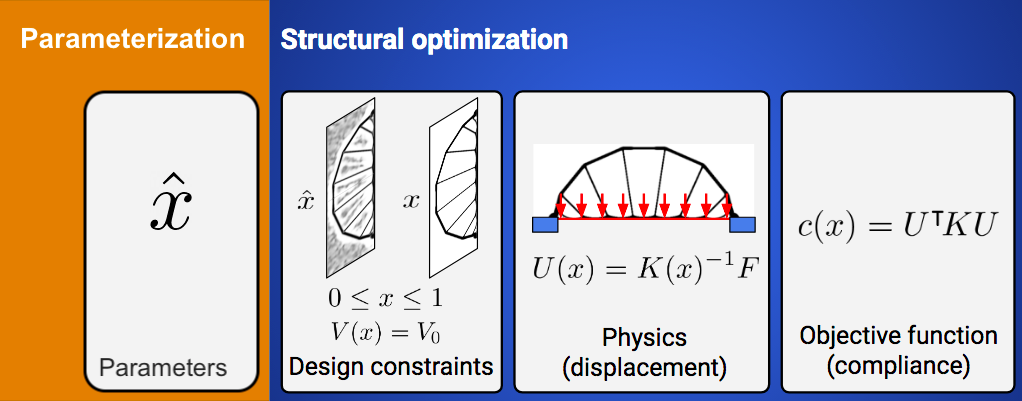Figure 7: Visualization of structural optimization. Here we are using it to design a bridge.

Automatic differentiation. The coolest thing about our implementation is that it is fully differentiable. In fact, we implemented everything in Autograd and then used automatic differentiation to solve for updates to the parameters. This made our code much simpler than previous approaches (which had implemented reverse-mode differentiation by hand).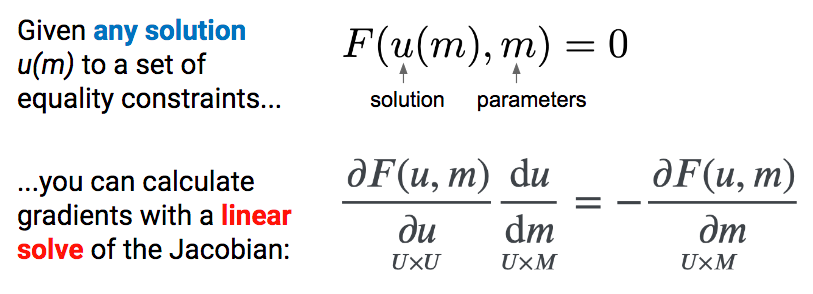Figure 8: Instead of differentiating directly through the root finder, we can use the implicit function theorem to differentiate through the optimal point.

The careful reader might be wondering how we differentiated through our root finder. At first we tried to naively backpropagate through the full search process. Bad idea. A better solution is to differentiate straight through the optimal point using implicit differentiation7 (Figure 7).

Reparameterizing the problem. Next, we built a CNN image generator in Keras and used it to reparameterize the grid of logits. The entire process, from the neural network forward pass to the constraint functions to the physics simulation, reduced to a single forward pass: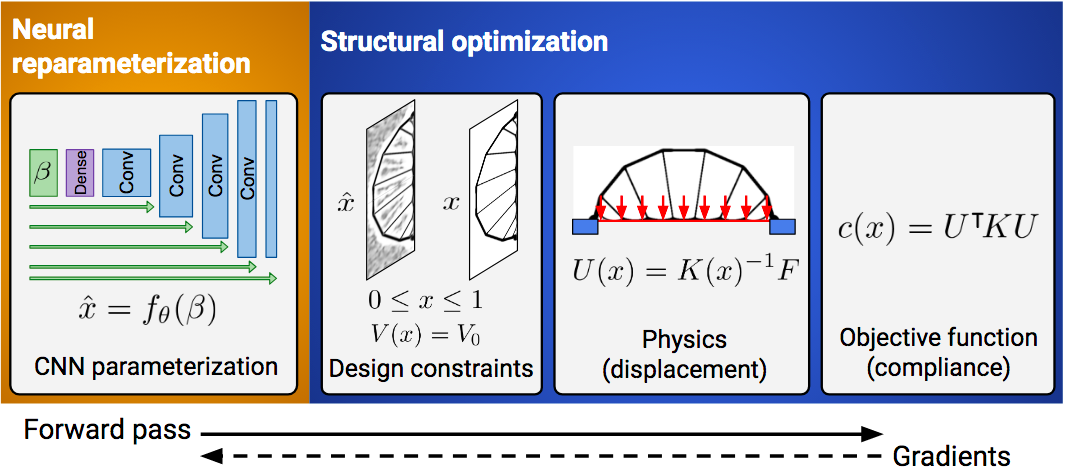Figure 9: Reparameterizing structural optimization. We implement the forward pass as a TensorFlow graph and compute gradients via automatic differentiation.

## Bridges, Towers, and Trees

In order to compare our method to baselines, we developed a suite of 116 structural optimization tasks. In designing these tasks, our goal was to create a distribution of diverse, well-studied problems with real-world significance. We started with a selection of problems from (Valdez et al. 2017)8 and (Sokol 2011).9 Most of these problems were simple beams with only a few forces, so we hand-designed additional tasks reflecting real-world designs such as bridges, towers, and trees.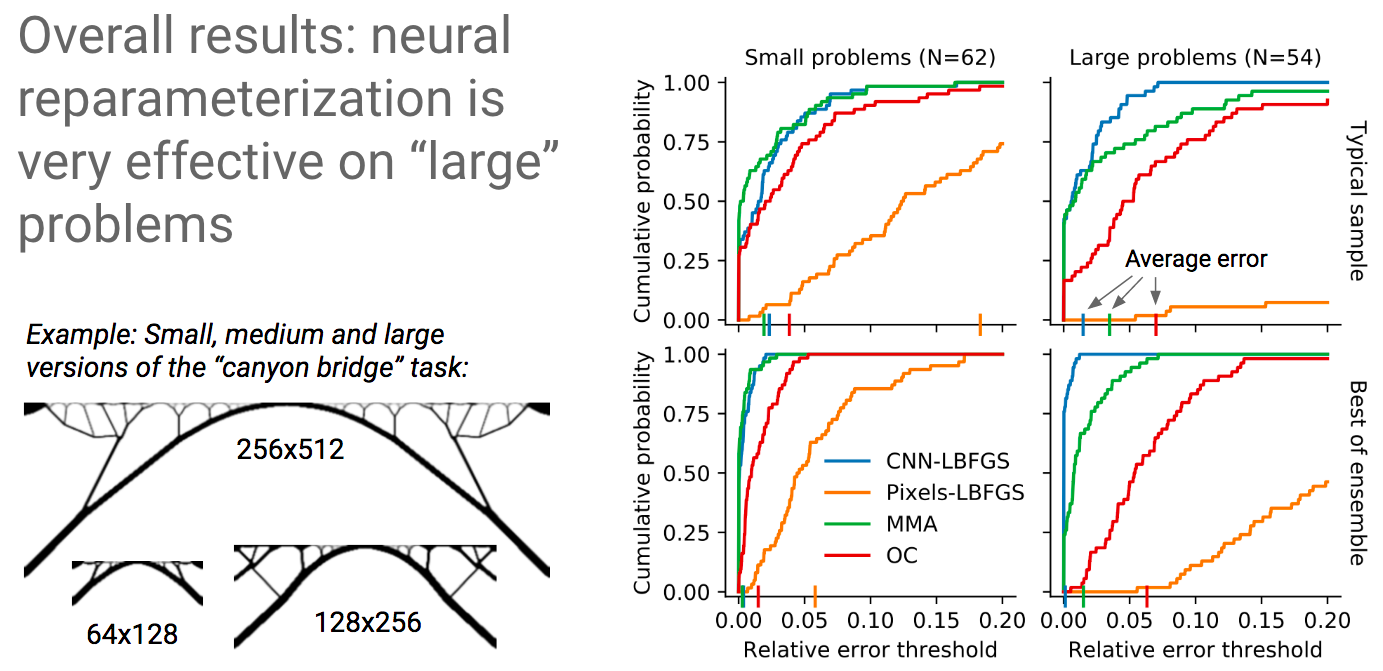Figure 10: Neural reparameterization improves structural optimization, especially for large problems.

Why do large problems benefit more? One of the first things we noticed was that large problems benefit more from our approach. Why is this? It turns out that finite grids suffer from a “mesh-dependency problem,” with solutions varying as grid resolution changes.10 When grid resolution is high, small-scale “spiderweb” patterns interfere with large-scale structures. We suspect that working in the weight space of a CNN allows us to optimize structures on several spatial scales at once, effectively solving the mesh-dependency problem. To investigate this idea, we plotted structures from all 116 design tasks and then chose five examples to highlight important qualitative trends (Figure 2).

One specific example is that the cantilever beam in Figure 2 had a total of eight supports under our method, whereas the next-best method (MMA11) used eighteen. Most of the qualitative results are at the beginning of this post, so refer to that section for more details.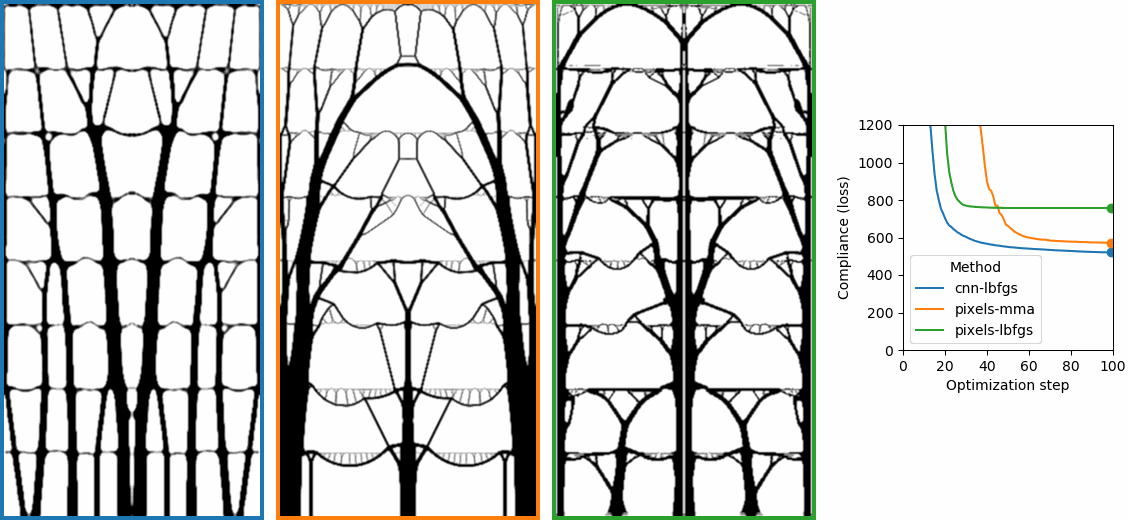Figure 11: Optimizing a multistory building. In the first frame, optimization happens in the weight space of a CNN. In the next two frames it happens on a finite element grid. Structures 2 and 3 are 7% and 54% worse.

## Closing thoughts

Structural optimization. This was a fun project because many of the results were beautiful and surprising. In fact, it convinced me that structural optimization is an undervalued tool for augmenting human creativity. With advances in 3D printing and fabrication, I hope it becomes more common in fields such as engineering and architecture.

Parameterization. A more general theme of this project is that parameterization matters much more than you might expect. We see this again and again. The most fundamental advances in deep learning – convolutional filters, forget gates, residual connections, and self-attention – should be thought of as advances in parameterization.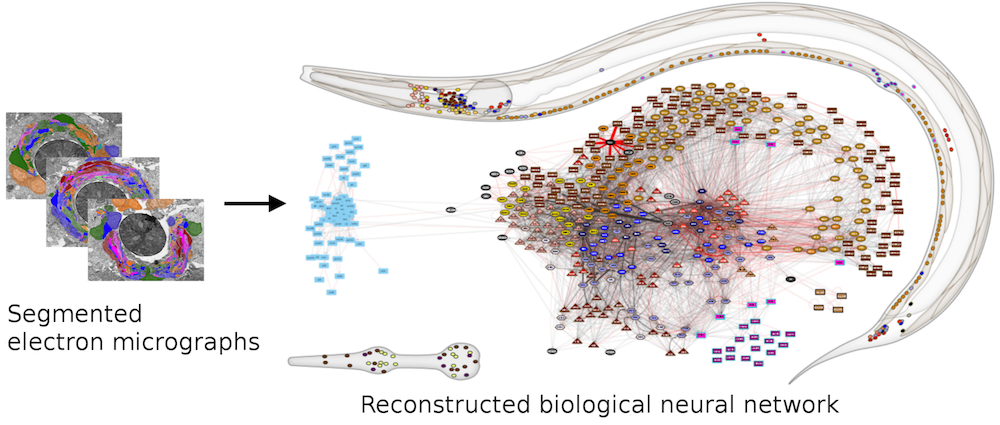Figure 12: Parameterization is important in biological systems as well. What sorts of priors might the C. elegans connectome encode?

This leads me to ask what other sorts of priors one could encode via parameterization. I’m particularly excited about a series of recent works that show how to encode complex, dataset-specific priors via network connectivity: “Weight Agnostic Neural Networks12”, “Lottery Tickets13,” and “Interaction Networks14”. There’s evidence that nature hard codes priors in a similar way. For example, a baby antelope can walk just a few minutes after birth, suggesting that this skill is hard-wired into the connectivity structure of its brain.

Takeaway. Regardless of whether you care about physics, deep learning, or biological analogies, I hope this post helped you appreciate the pivotal role of parameterization.

1. Ulyanov, Dmitry, Andrea Vedaldi, and Victor Lempitsky. Deep Image Prior. Proceedings of the IEEE Conference on Computer Vision and Pattern Recognition. 2018.

2. Mordvintsev, Alexander, et al. Differentiable Image Parameterizations. Distill 3.7 (2018): e12.

3. Azadi, S., Fisher, M., Kim, V. G., Wang, Z., Shechtman, E., and Darrell, T. Multi-content GAN for few-shot font style transfer. In The IEEE Conference on Computer Vision and Pattern Recognition (CVPR), June 2018.

4. Zhu, Y., Zabaras, N., Koutsourelakis, P.-S., and Perdikaris, P. Physics-constrained deep learning for high-dimensional surrogate modeling and uncertainty quantification without labeled data. Journal of Computational Physics, 394:56–81, 2019.

5. Dittmer, S., Kluth, T., Maass, P., and Baguer, D. O. Regularization by architecture: A deep prior approach for inverse problems. Preprint, 2018.

6. Andreassen, E., Clausen, A., Schevenels, M., Lazarov, B. S., and Sigmund, O. Efficient topology optimization in MATLAB using 88 lines of code. Structural and Multidisciplinary Optimization, 43(1):1–16, 2011.

7. Griewank, A. and Faure, C. Reduced functions, gradients and hessians from fixed-point iterations for state equations. Numerical Algorithms, 30(2):113–139, 2002.

8. Valdez, S. I., Botello, S., Ochoa, M. A., Marroquín, J. L., and Cardoso, V. Topology optimization benchmarks in 2D: Results for minimum compliance and minimum volume in planar stress problems. Arch. Comput. Methods Eng., 24(4):803–839, November 2017.

9. Sokol, T. A 99 line code for discretized Michell truss optimization written in Mathematica. Structural and Multidisciplinary Optimization, 43(2):181–190, 2011.

10. Sigmund, O. and Petersson, J. Numerical instabilities in topology optimization: A survey on procedures dealing with checkerboards, mesh-dependencies and local minima. Structural optimization, 16:68–75, 1998.

11. Svanberg, K. The method of moving asymptotes-a new method for structural optimization. International Journal for Numerical Methods in Engineering, 24(2):359–373, 1987.

12. Gaier, Adam, and David Ha. Weight Agnostic Neural Networks. Neural Information Processing Systems (2019).

13. Frankle, Jonathan, and Michael Carbin. “The lottery ticket hypothesis: Finding sparse, trainable neural networks.” ICLR 2019.

14. Battaglia, Peter, et al. “Interaction networks for learning about objects, relations and physics.” Advances in neural information processing systems. 2016.